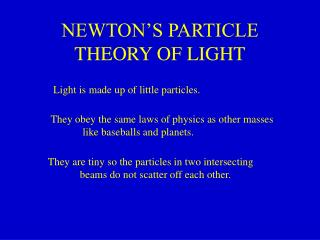DownloadDownload PresentationNEWTON’S PARTICLE THEORY OF LIGHT

# NEWTON’S PARTICLE THEORY OF LIGHT

Download Presentation## NEWTON’S PARTICLE THEORY OF LIGHT

- - - - - - - - - - - - - - - - - - - - - - - - - - - E N D - - - - - - - - - - - - - - - - - - - - - - - - - - -
##### Presentation Transcript

1. NEWTON’S PARTICLE THEORY OF LIGHT Light is made up of little particles. They obey the same laws of physics as other masses like baseballs and planets. They are tiny so the particles in two intersecting beams do not scatter off each other.

2. PARTICLE THEORY OF REFRACTION A light particle deep within a medium experiences no net force. Near an interface, e.g. between air and water, light particles experience an attractive force towards the water. Could this be the cause of refraction?

3. vpar vpar qi vair air water qr vwater

4. NEWTON’S EXPLANATION OF SNELL’S LAW sin(qi) = vpar/vair sin(qr) = vpar/vwater sin(qi)/sin(qr) = vwater/vair

5. OTHER PROPERTIES Colors Polarization

6. water-filled tube Decisive Test of Particle Theory fixed mirror source rotating mirror air water

7. WAVE MOTION A wave is a pattern, or shape, or disturbance, traveling through a medium. Examples: Sound is a pressure wave in air. Sideways vibration of stretched string. Football stadium “wave”.

8. TORSIONAL WAVES Waves reflect from ends in this example also.

9. FASTER TORSIONAL WAVES

10. SUPERPOSITION When two wave amplitudes occur at the same point, they simply add. They do not scatter from each other. Example: Torsion waves passing through each other.

11. v l T PERIODIC WAVES

12. PERIODIC WAVE SPEED T = period of rope motion. f = frequency = 1/T v = wave speed Wavelength = l = distance between wave crests Basic relation: v = distance moved/time = l/T = fl Example: WTJU frequency = 91 MHz. l = c/f = 3*108/0.9*108 = 3.3m

13. r lr REFRACTION OF WAVES li i sin(i)/sin(r) = li/lr = fli/flr = vi/vr

14. REFRACTION fast slow fast

15. INTERFERENCE Constructive Interference: Destructive Interference

16. YOUNG TWO-SLIT INTERFERENCE PATTERN Bright Dim Bright Dim path difference l

17. SOAP FILMS film thickness = t path difference ~ 2t and varies as film drains and thins. Colored horizontal fringes can be seen.

18. COLOR AND WAVELENGTH Color Wavelength (nm) Red 650 Yellow 580 Green 540 Blue 470 Violet 440